## The motion of a set of particles moving along the x-axis is governed by the differential equation dx dt = t 3 – x3, where x1t2 denotes the p

Question

The motion of a set of particles moving along the x-axis is governed by the differential equation dx dt = t 3 – x3, where x1t2 denotes the position at time t of the particle. (a) If a particle is located at x = 1 when t = 2, what is its velocity at this time? (b) Show that the acceleration of a particle is

in progress 0
1 week 2021-09-05T08:05:59+00:00 1 Answers 0 views 0

a)V=7 m/s

b)a=3t²-3x² t³ +3 x ⁵

Explanation:

Given that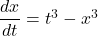a)

We know that velocity V is given as follows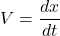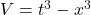At t= 2 s and x= 1 m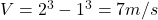V=7 m/s

b)

Acceleration a is given as follows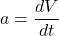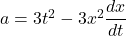Now by putting the values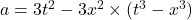a=3t²-3x² t³ +3 x ⁵

Therefore the acceleration of a particle will be 3t²-3x² t³ +3 x ⁵.